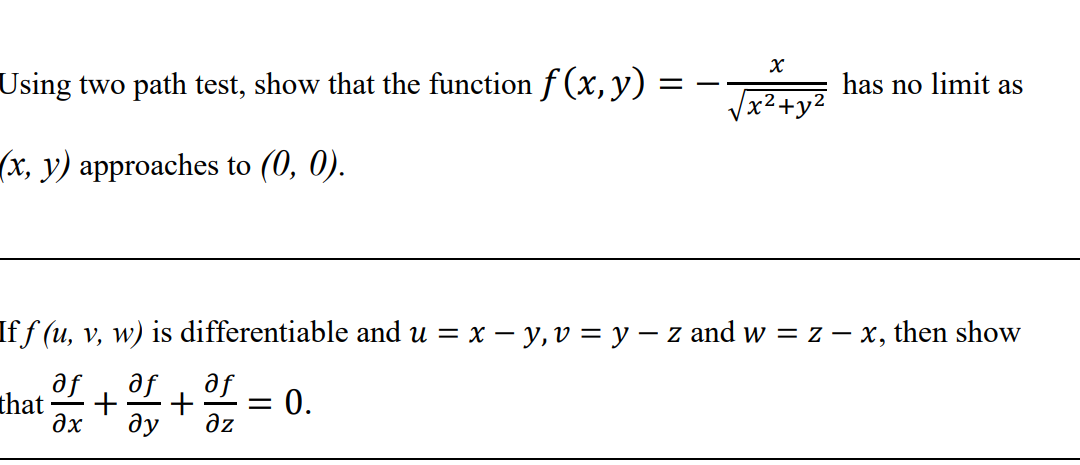### Create an Account

Home / Questions / х Using two path test, show that the function f(x,y) has no limit as x2+y? (x, y) approach...

# х Using two path test, show that the function f(x,y) has no limit as x2+y? (x, y) approaches to (0, 0). Iff (u, v, w) is differentiable and u = x – y, v = y – z and w = 2 – x, then show af that + + =

х Using two path test, show that the function f(x,y) has no limit as x2+y? (x, y) approaches to (0, 0). Iff (u, v, w) is differentiable and u = x – y, v = y – z and w = 2 – x, then show af that + + = 0. ax ду дz af of -May 17 2021 View more View LessSubscribe To Get Solution